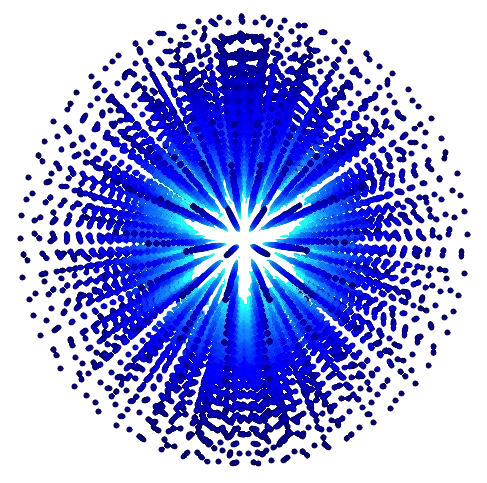# FLAG: Exact Fourier-Laguerre transform on the ballFLAG provides a fast implementation of the Fourier-Laguerre transform, a novel 3D transform exploiting an exact quadrature rule on the ball to construct an exact harmonic transform in 3D spherical coordinates.

The angular part of the Fourier-Laguerre transform uses the MW sampling theorem and the exact spherical harmonic transform implemented in the SSHT code.

The radial sampling scheme arises from an exact quadrature on the radial half-line using damped Laguerre polynomials. The radial transform can in fact be used to compute the spherical Bessel transform exactly, and the Fourier-Laguerre transform is thus closely related to the Fourier-Bessel transform.